Email us to get an instant 20% discount on highly effective K-12 Math & English kwizNET Programs!

Online Quiz (WorksheetABCD)

Questions Per Quiz = 2 4 6 8 10

High School Mathematics - 26.7 Inverse Matrix

 Method:- 1. Check whether the given matrix 'A' is a singular or non-singular matrix. That is |A| � 0. 2. If A is singular, A-1 does not exit. If A is non-singular proceed to find the inverse of the gives matrix. 3. Interchanging the elements of the principal diagonal of A. 4. Change the sign of the other two elements in A. 5. Multiply the resultant matrix with the scalar 1/(ad-bc).Directions: Find the inverse of the given matrix. Also write at least ten examples of your own.
 Q 1: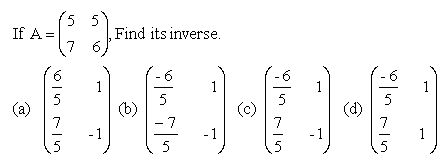cbda Q 2: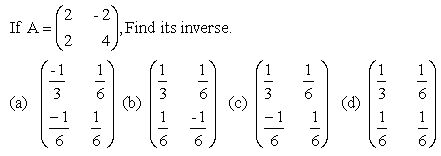abcd Q 3:cadb Q 4: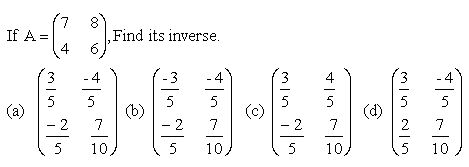abcd Q 5: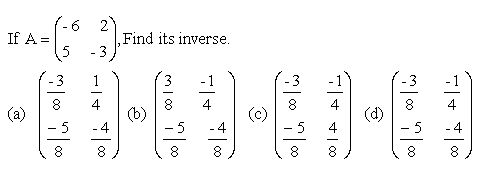none of the choicesbca Q 6: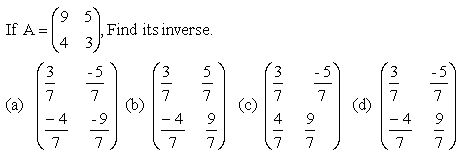cbad Question 7: This question is available to subscribers only! Question 8: This question is available to subscribers only!